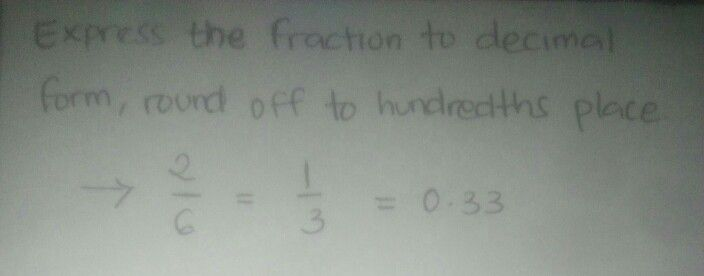Symbol
Problembora bne Herlasd diod bavsla 20 tedyshiov bns lediesd diod bsvsla TEST $53$ Expressing Rational Numbers from Fraction Form to Decimal Form and $Mlce-versa$ $A$ Express the Fractions to Decimals forms, Round off your answers to hundredths place. $1.\right)$ $2/6=$ $0.P0$ $f0$ $1$ $C$ $-31$ $5Q.\left(=3$ $S$ $\sum$ $2$